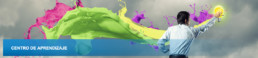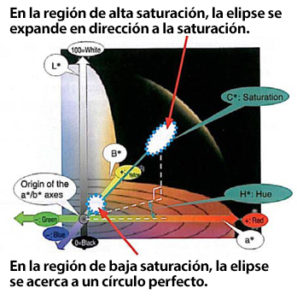# Fórmula CIE 2000 para diferenciar colorFeature CIE 2000 color difference formula was developed to solve the problem of the differences in the evaluation between color meters and the human eye caused by the difference in the shape and size of the color discrimination threshold of the human eye.

The CIE 2000 color difference formula is not an attempt to build a color space in which of the color discrimination threshold of the human eye are uniform. Instead, it defines a calculation so that the color difference calculated by color meters become close to the color discrimination threshold of the human eye on the solid color space of CIE Lab (L*a*b* color space). Specifically, weigh is assigned to the lightness difference ΔL*, saturation difference ΔC*, and hue difference ΔH* by using weigh coefficients SL, SC, and Sh respectively. These weighting coefficients SL, SC, and Sh include the effects of lightness L*, saturation C*, and hue angle H. Consequently, the calculation incorporates the characteristics of the color discrimination threshold of the human eye on the color space of CIE Lab (L*a*b* color system): 1) Saturation dependency, 2) Hue dependency, and 3) Lightness dependency.

With the CIE Lab (L*a*b* color space) color difference formula, the color difference evaluation ranges represented by color difference ΔE*ab and chromaticity index difference Δa*b* were a perfect circle or a square in the L*a*b* solid color space. With CIE 2000, color difference ΔE00 is represented with an ellipse having the major axis in the direction of saturation, which is similar to the shape of the color discrimination threshold of the human eye. In the region with lower saturation, the weight coefficients SL, SC, and Sh approach 1 respectively, making the ellipse more circular.

In the region with higher saturation, the weighing coefficient SC becomes greater compared with other coefficients SL and Sh so that the ellipse becomes longer in the direction of saturation (lower sensitivity to saturation differences).

With the CIE 2000 color difference formula, the effect on the hue angle is also considered. As a result, the formula can cope with another characteristics of the color discrimination threshold of the human eye on the color space of CIE Lab (L*a*b* color space): 4) Change in the direction of the color discrimination threshold around the hue angle of 270 degrees (blue) (deviation from the direction of saturation).